In this lesson we will review simple regression and demonstrate a linear regression model in R. This should be a review of regression from MA206. You can also take a look at your text book pages 143-151 to get a more detailed description of linear regression.

## Import and Explore Cars Data

We’re going to use some data about various car models in order to refresh our memories on the basics of regression. You can import this data with the following command

cars<-read.csv("cars.csv",as.is=TRUE)  ##Remember, the as.is=TRUE means that text data becomes character data 

Here’s a description of what each of the fields in this data set represent

Name Description
mpg Miles/(US) gallon
cyl Number of cylinders
disp Displacement (cu.in.)
hp Gross horsepower
drat Rear axle ratio
wt Weight (lb/1000)
qsec 1/4 mile time
vs V/S
am Transmission (0 = automatic, 1 = manual)
gear Number of forward gears
carb Number of carburetors

## Pairs Plot

The pairs plot is a very powerful plot that helps a data scientist explore data. This is definitely not available to you in Excel. The pairs plot is used to plot all numeric variables against each other. You can only produce a pairs plot on numeric variables in your data set. The primary variable we are interested in the cars data is miles per gallon. We will start by comparing mpg to the first five variables.

pairs(cars[,2:7])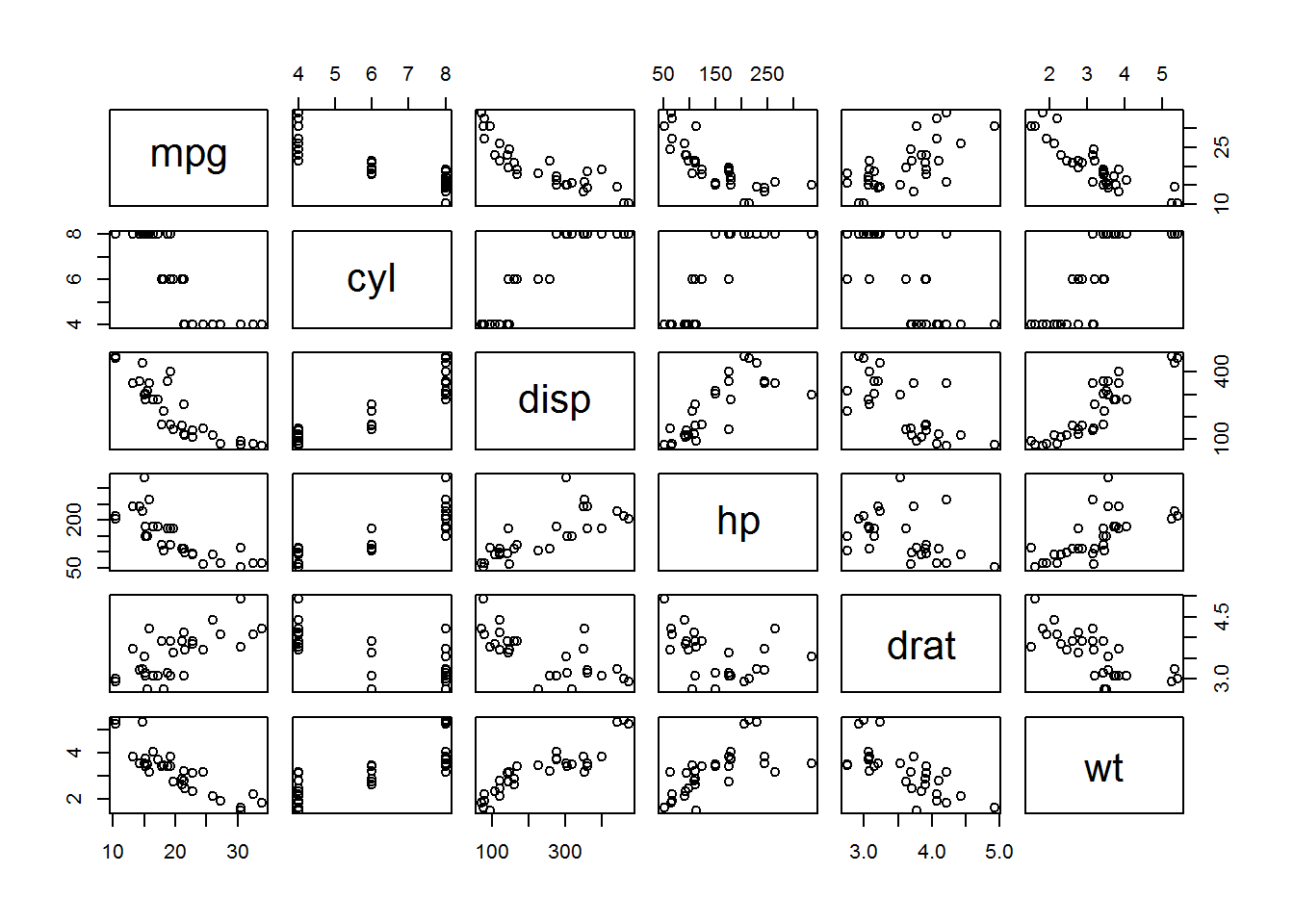Now we compare mpg to the last five variables:

pairs(cars[,c(2,8:12)])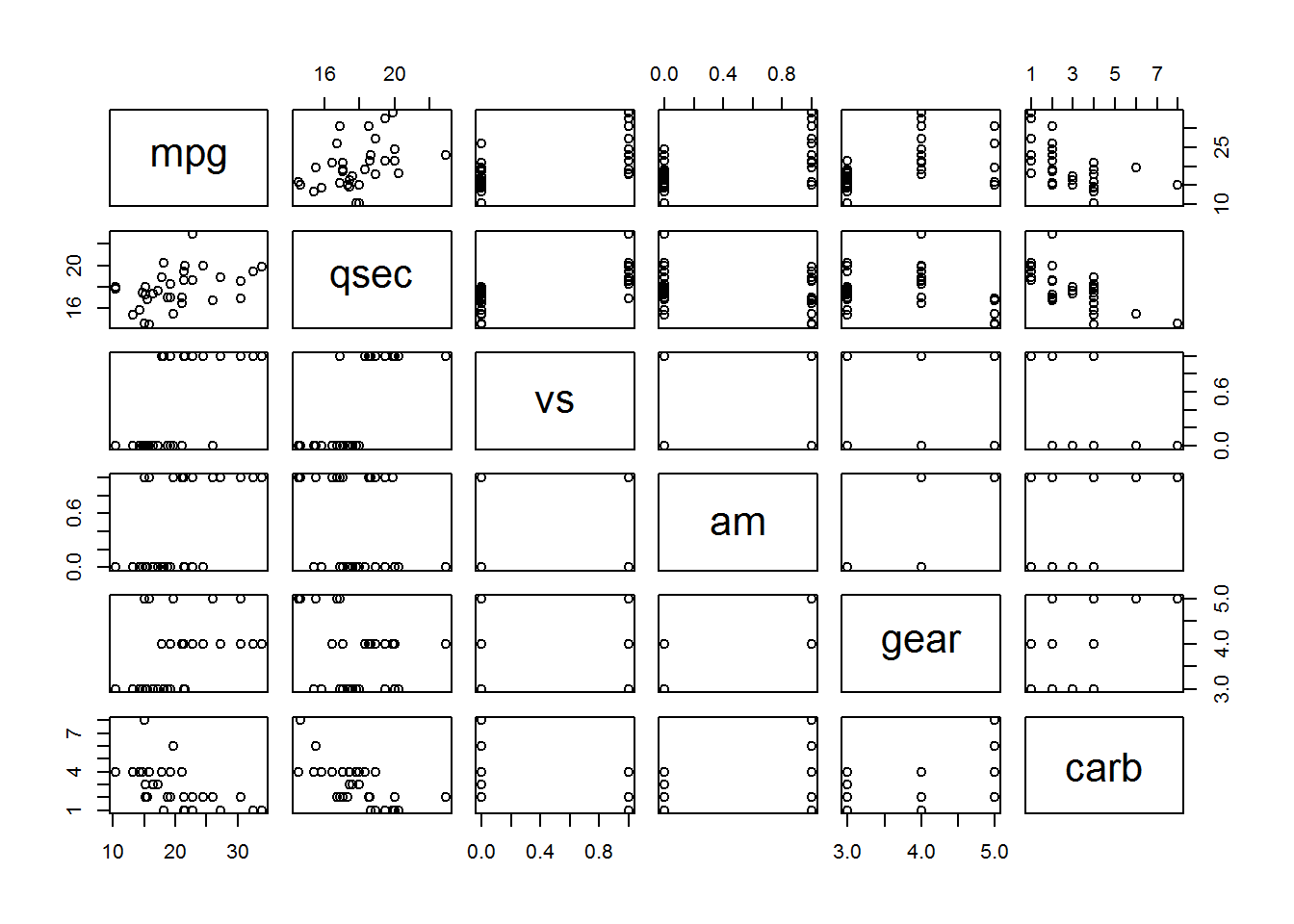From these two plots, we see that there is definitely a relationship between mpg and disp, hp, drat, wt, and carb.

## Review of Simple Linear Regression

Before we get into regression, let’s try to remember what correlation is. Correlation measure association or dependency quantitatively. The formal mathematical equation for correlation is:

$r=\frac{1}{(n-1)}\sum\limits_{i=1}^{n}\left( \frac{(x_i-\bar{x})}{s_x}\right)\left( \frac{(y_i-\bar{y})}{s_y}\right)$

It’s a good thing you won’t ever have to use this equation in SE350. Instead, you will either use the CORREL() command in Excel or the cor command in R. The following list helps us remember the basics of correlation:

• Between -1 and 1
• $$R >0$$ indicates positive relationship
• $$R<0$$ indicates negative relationship
• When r is close to 1 or negative 1, the association is strong, but close to 0 indicates a weak association

If we wanted to measure the correlation between miles per gallon and displacement, we would use the following command in R:

cor(cars$mpg,cars$disp)  ##It does not matter what order you put the two variables in this function
##  -0.8476

We see that there is a strong negative relationship between miles per gallon and displacement.

The process of using data to formulate relationships is known as regression analysis.

• Response Variable: the variable that will be predicted by the values of other variables
• Explanatory Variables: These variables can explain quantitatively, at least in part, the values of the the response variable.
• Simple Regressions: One explanatory variable (this lesson)
• Multiple Regressions: More than one explanatory variable (next lesson)

Regressions models can fall into two other categories: Linear (SE350) or Nonlinear (Not SE350).

Regression is a means to find the line that most closely matches the observed relationship between x and y. $y=a+bx+e$

Most common approach is the sum of squared differences between the observed and model values. This is what is going on “underneath the hood” of your computer when you do regression:

$e_i=y_i-y=y_i-(a+bx_i)$ $SS=\sum\limits_{i=1}^{n}e_{i}^{2}=\sum\limits_{i=1}^{n}(y_i-a-bx_i)^2$

All that this equation is doing is placing the linear line in such a way that it minimizes the sum of the square of the error lengths (see picture of errors below):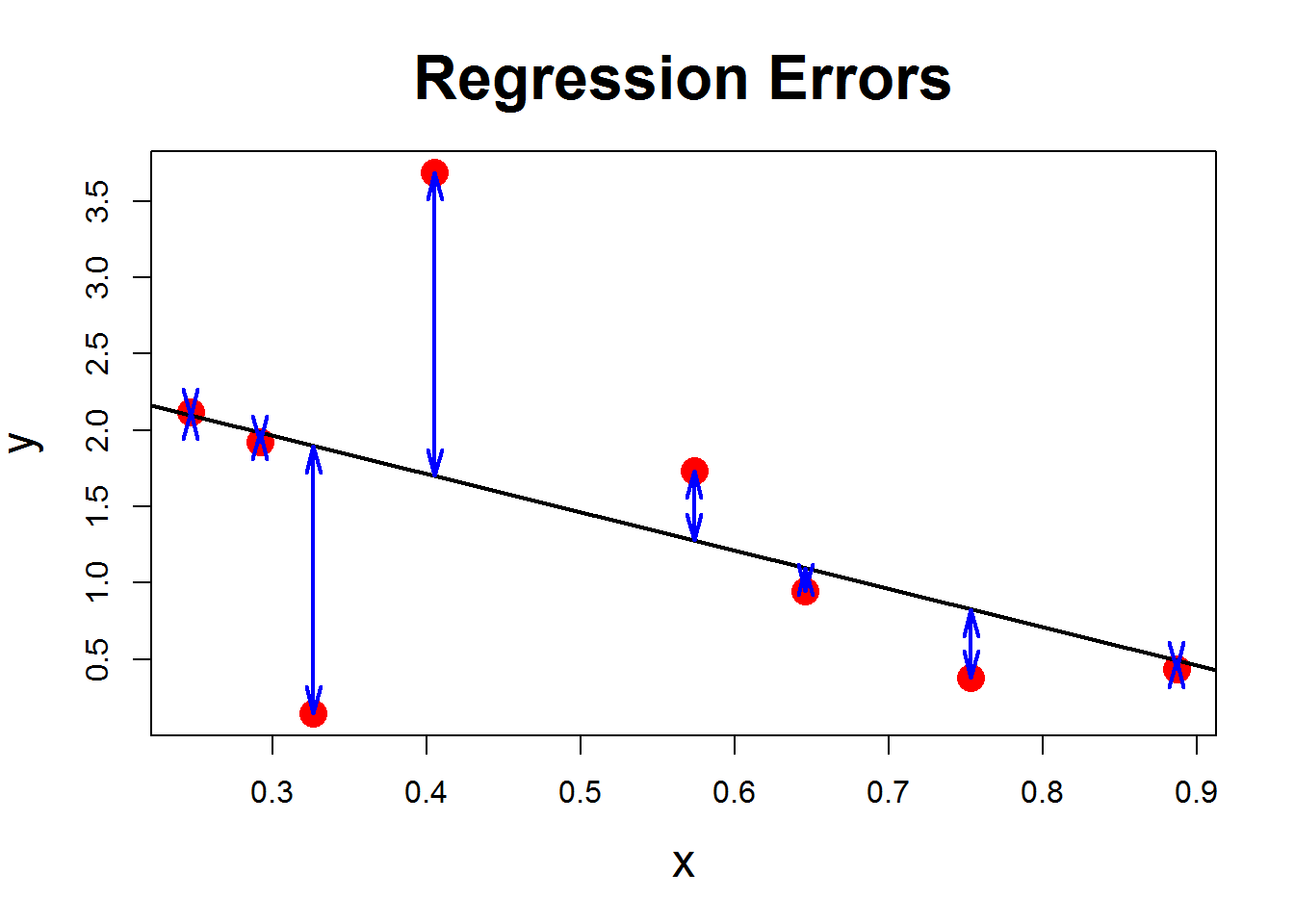## Basic Model

The equation below builds a linear regression model for the cars data with mpg and disp. Notice that all you have to do is input the formula with the response variable on the left side of the ~ and the explanatory variable on the right side. This makes it extremely easy to choose any numeric variable in your data frame.

cars.lm<-lm(mpg~disp,data=cars)   ##Note that lm stands for linear model

## Summary of Model

Just running the model doesn’t tell us anything. To get the basic summary of the linear model, we use the command below:

summary(cars.lm)
##
## Call:
## lm(formula = mpg ~ disp, data = cars)
##
## Residuals:
##    Min     1Q Median     3Q    Max
## -4.892 -2.202 -0.963  1.627  7.231
##
## Coefficients:
##             Estimate Std. Error t value Pr(>|t|)
## (Intercept) 29.59985    1.22972   24.07  < 2e-16 ***
## disp        -0.04122    0.00471   -8.75  9.4e-10 ***
## ---
## Signif. codes:  0 '***' 0.001 '**' 0.01 '*' 0.05 '.' 0.1 ' ' 1
##
## Residual standard error: 3.25 on 30 degrees of freedom
## Multiple R-squared:  0.718,  Adjusted R-squared:  0.709
## F-statistic: 76.5 on 1 and 30 DF,  p-value: 9.38e-10

Let’s start by looking at the Coefficients. Note that the intercept is 37.285 and the coefficient of weight is -5.344. Remember that the coefficient for weight is the slope of our regression line (in other words, this says that you will lose roughly 5 miles per gallon in fuel economy for every additional 1000 lbs of weight). The equation of our regression line is

$y=37.285-5.344x$

The last number in the coefficients table, with the title Pr(>|t|), is asking the question How likely is it that we would get an estimate of the regression coefficient at least this large if the true value of the regression coefficient were zero? In other words, what is the chance that our intercept or our slope were actually zero. This is the probability that this coefficient is not significant. The smaller this number is, the more significant the coefficient is in our linear model. This will be used more in our next class when we begin having more than one explanatory variable.

The bottom three lines of the summary have several statistics in them:

• $$R^2$$: Measures the percent of variation in the response variable accounted for by the regression model (note that this is the square of correlation)
• F-statistic and its significance: How likely is it that we would get the $$R^2$$ value that we observed if ALL of the true regression coefficients were zero?
• Adjusted $$R^2$$: This is another form of $$R^2$$ that has been adjusted if there are more than one explanatory variable. It is only considered in mutiple variable regression (next lesson).

## Plotting Linear Regression

We can graph our data and linear regression line with the following command:

plot(cars$disp,cars$mpg,xlab="disp",ylab="mpg",pch=16,main="Linear Regression with mpg~disp")
abline(cars.lm,col="red")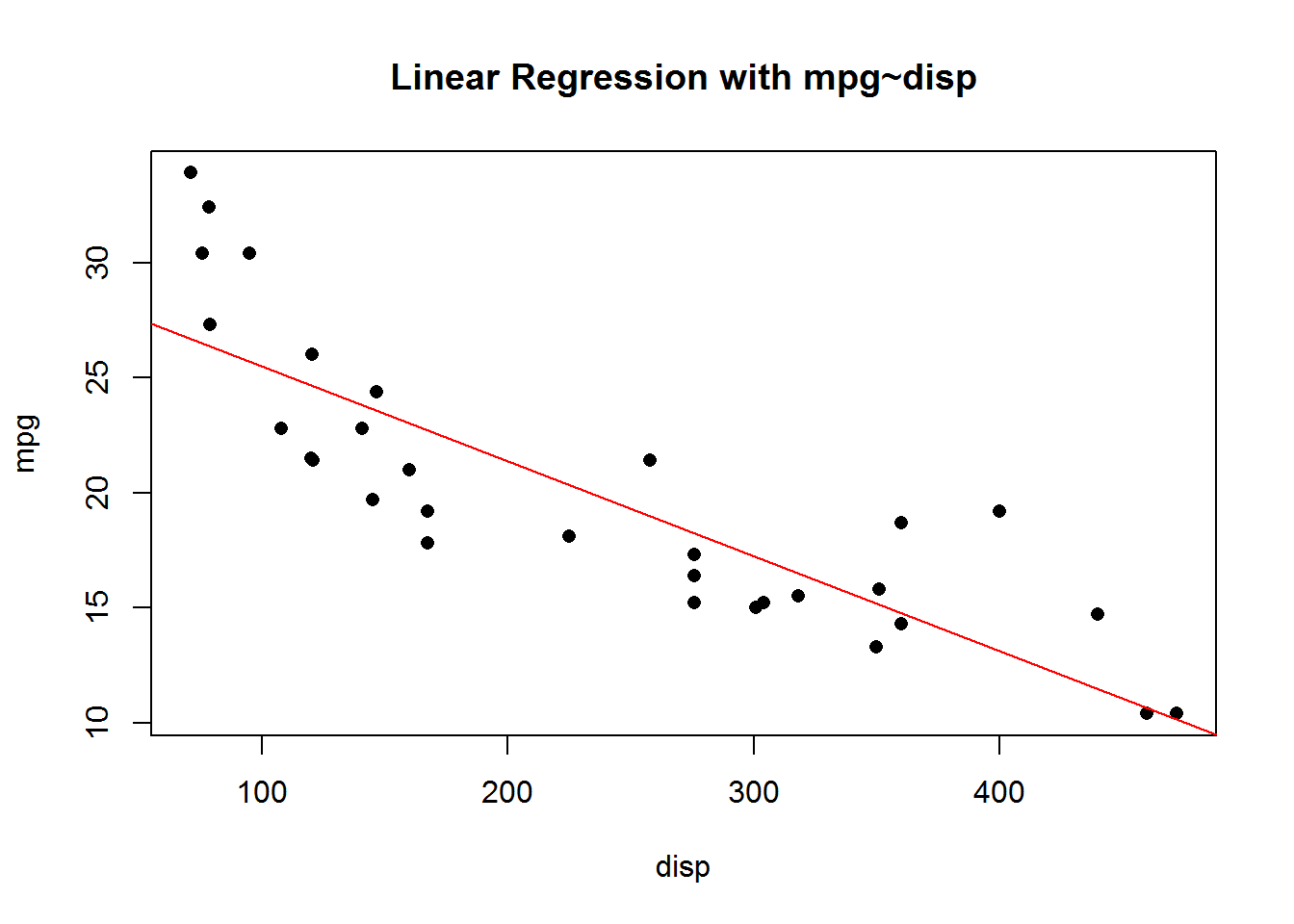Notice that overall we have a good fit. It does look like our data is a little non-linear, however.

## Assumptions of Linear Regression

1. The relationship between the independent (X) and the dependent (Y) variables is linear.
2. Errors in prediction of the value of Y are distributed in a way that approaches the normal curve.
3. Errors in prediction of the value of Y are all independent of one another.
4. The distribution of the errors in prediction of the value of Y is constant regardless of the value of X (constant variance).

We can test our assumptions with the following plot command:

plot(cars.lm)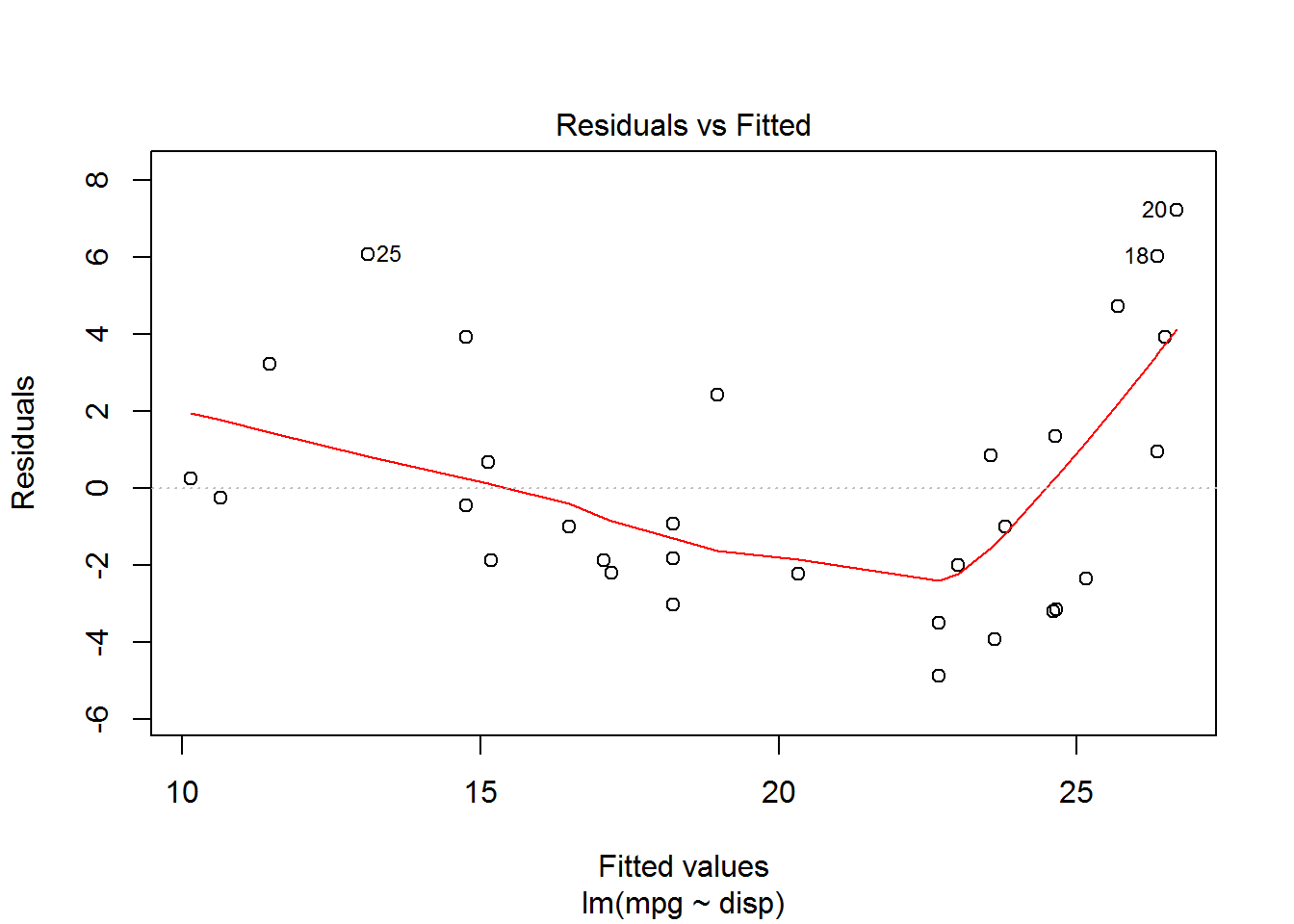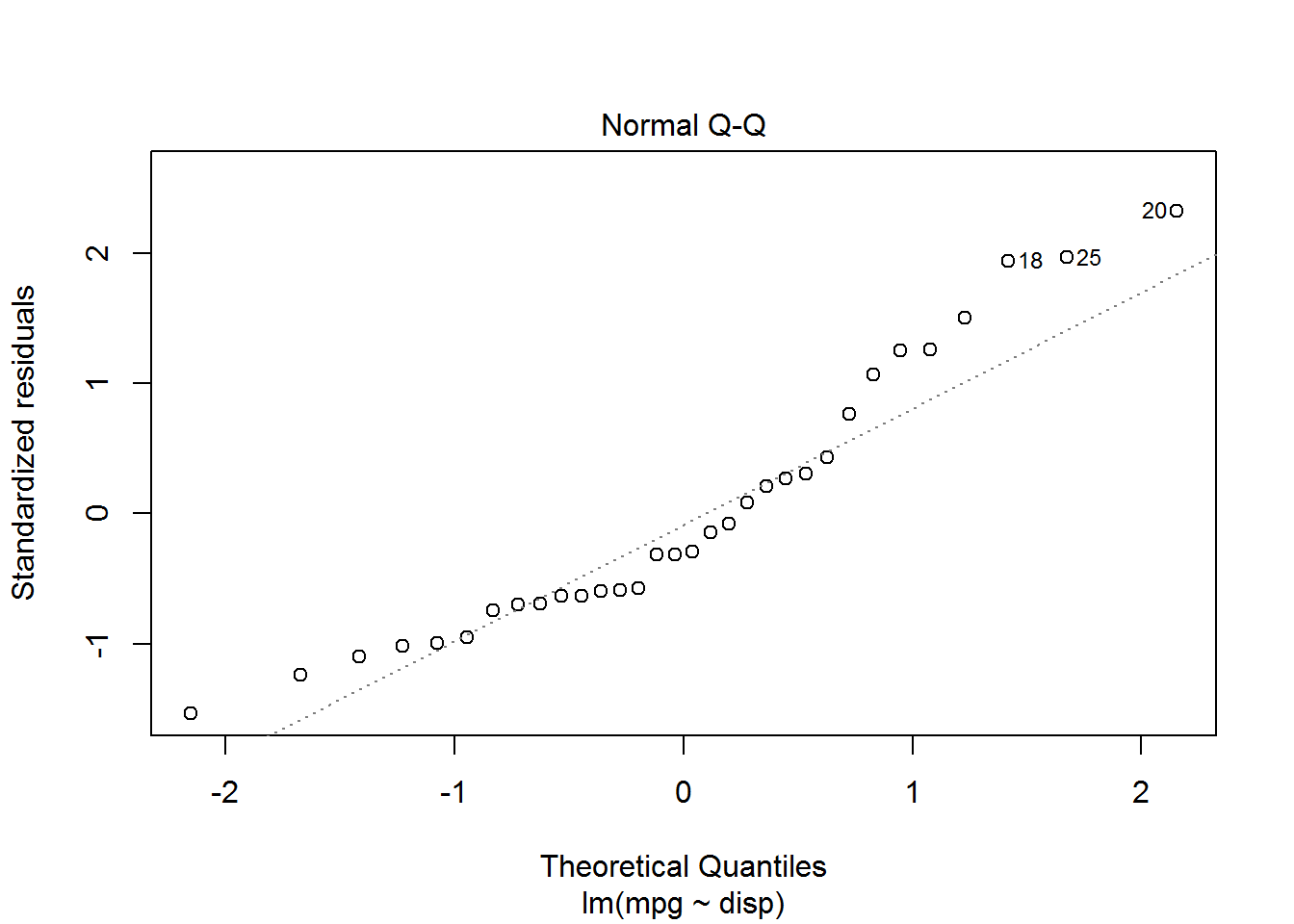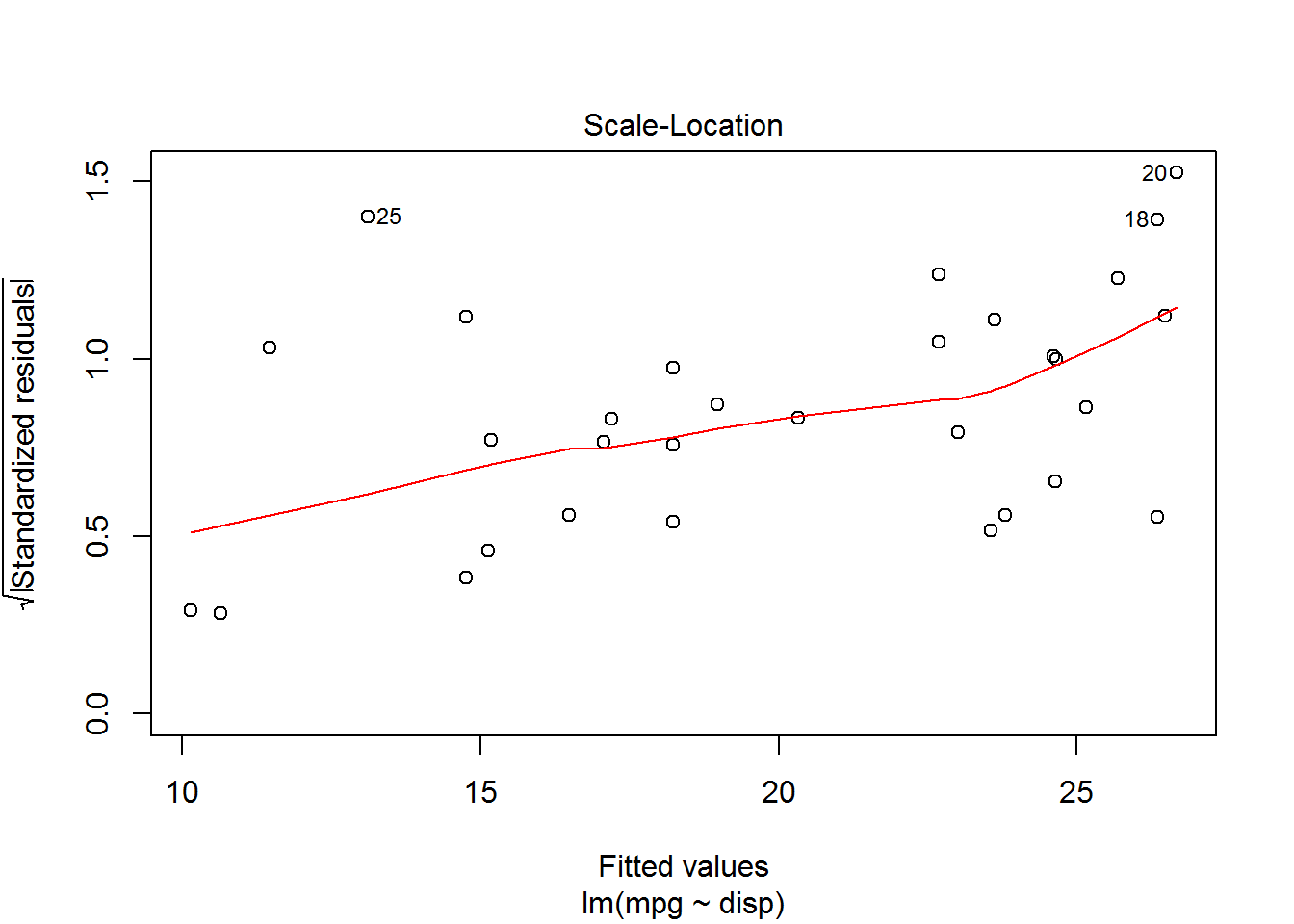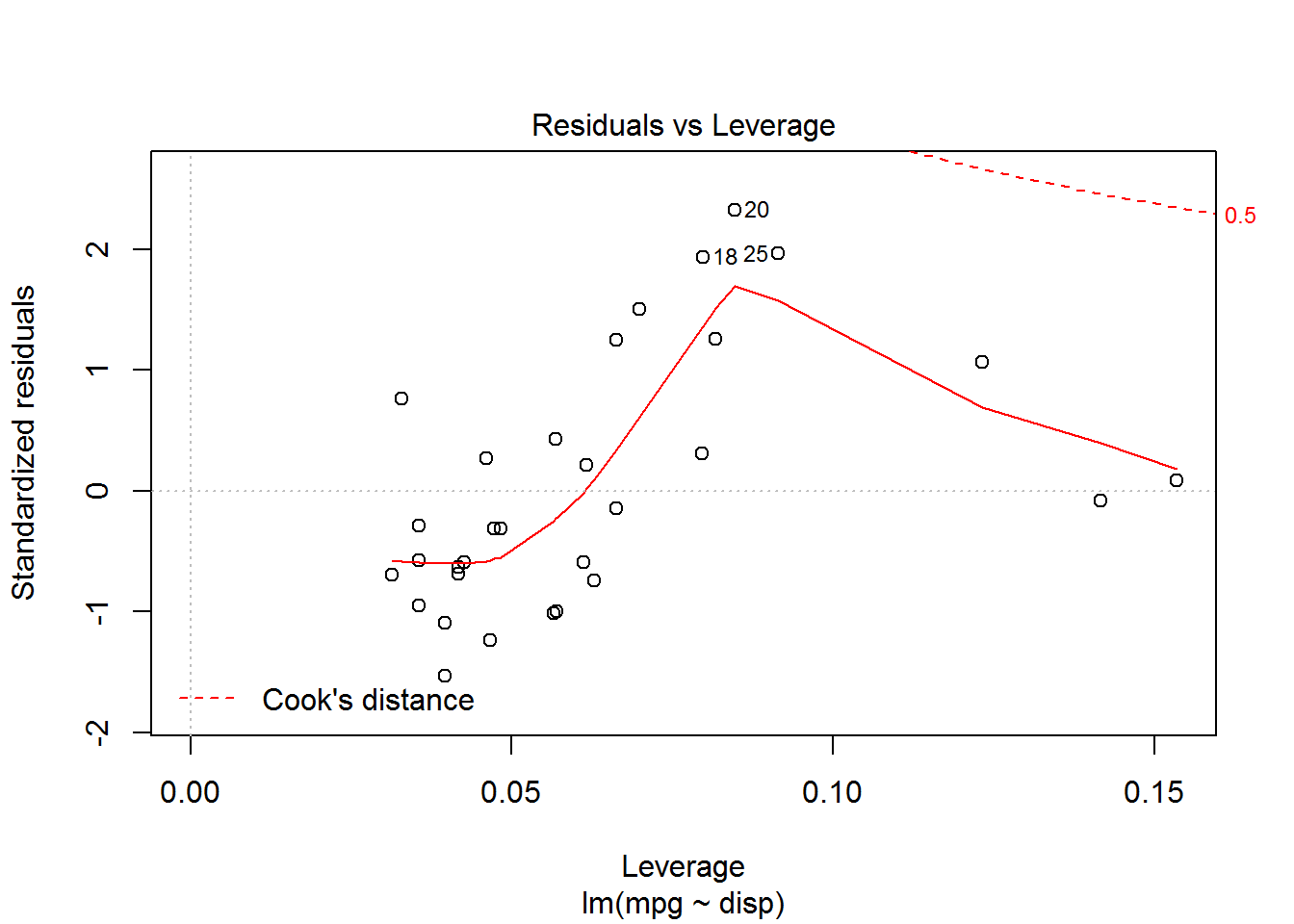The first plot (Residuals vs Fitted) will allow us to check assumptions 1 (linearity) and 4 (equal variance). Here’s a helpful figure to use in evaluating this plot: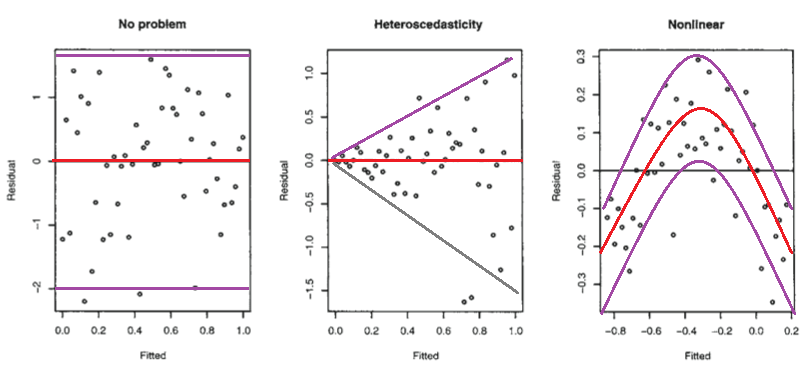If the pattern of the first plot is an “alligator mouth”, then you have a problem with unequal variance, which is statically called heteroscedasticity. If the red line is not fairly straight and horizontal, then you have a problem with non-linearity. Notice that our model does have non-linearity.

The second plot (Normal Q-Q plot) allows us to assess the normality of the residuals. If the points are pretty close to the dotted line, then the residuals approximate a normal distribution.

In the last plot (Residuals vs Leverage), if you have any of your points that are outside of Cook’s distance (i.e. outside of the red dotted line), then that point is an extreme outlier and will have a negative affect on your regression results.

## Finding the Best Predictive Statistic

Don’t always go with you first guess for an explanatory variable. R allows you to quickly explore more of your variables to see if these provide a better “explanation” of miles-per-gallon. Let’s try weight.

cars.lm2<-lm(mpg~wt,data=cars)
summary(cars.lm2)
##
## Call:
## lm(formula = mpg ~ wt, data = cars)
##
## Residuals:
##    Min     1Q Median     3Q    Max
## -4.543 -2.365 -0.125  1.410  6.873
##
## Coefficients:
##             Estimate Std. Error t value Pr(>|t|)
## (Intercept)   37.285      1.878   19.86  < 2e-16 ***
## wt            -5.344      0.559   -9.56  1.3e-10 ***
## ---
## Signif. codes:  0 '***' 0.001 '**' 0.01 '*' 0.05 '.' 0.1 ' ' 1
##
## Residual standard error: 3.05 on 30 degrees of freedom
## Multiple R-squared:  0.753,  Adjusted R-squared:  0.745
## F-statistic: 91.4 on 1 and 30 DF,  p-value: 1.29e-10

This has an $$R^2$$ of 0.75, compared to 0.71 in first model. This model using weight therefore has a better fit and is a slightly stronger model.

A plot of this model is given below:

plot(cars$wt,cars$mpg,xlab="wt",ylab="mpg",pch=16,main="Linear Regression with mpg~weight")
abline(cars.lm2,col="red")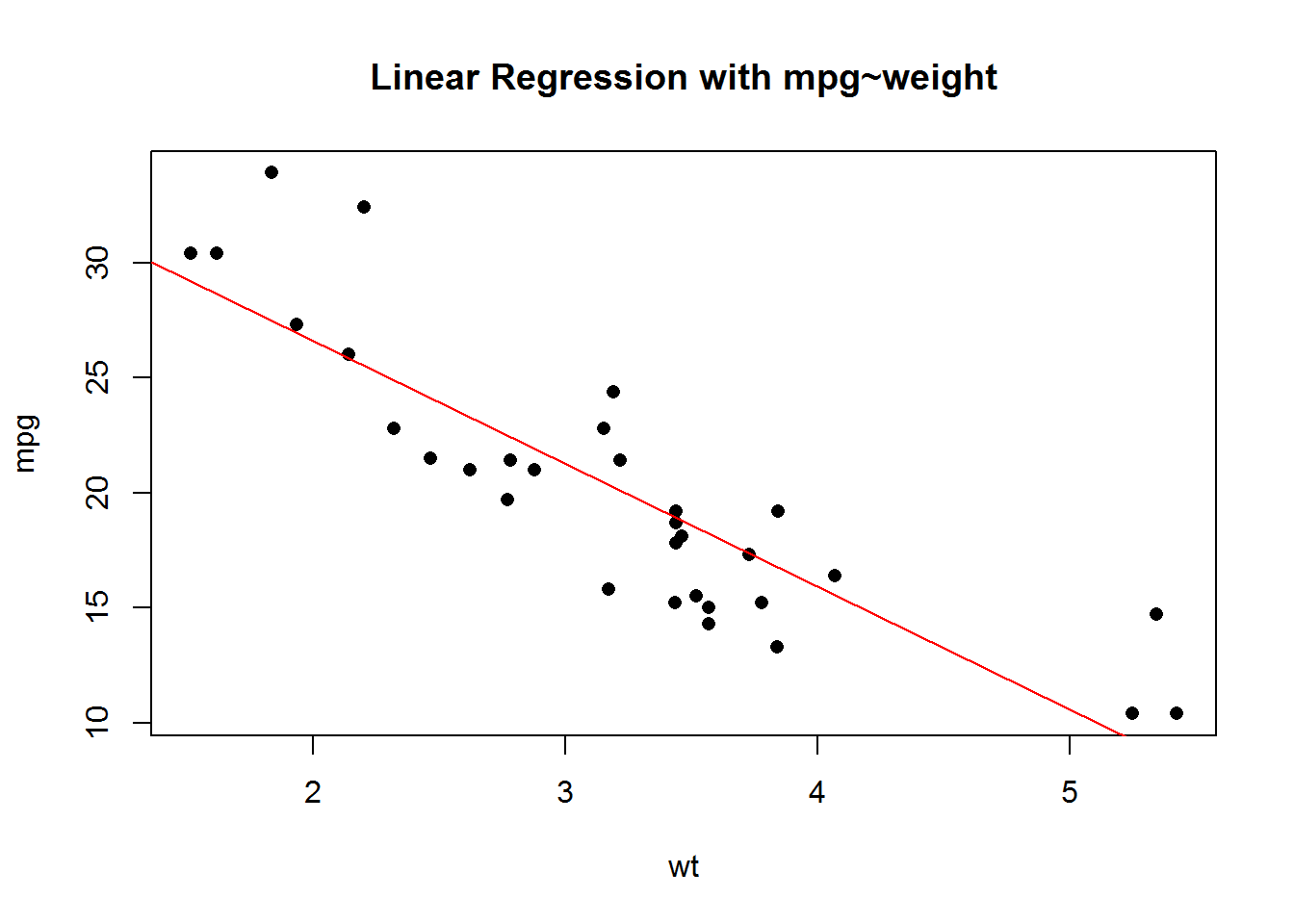Check the assumptions for this model. You will see that this model has slightly better linearity, the residuals are more normal, but there is an extreme outlier(the Chrysler Imperial).

## Predicting

To predict with our linear model, you will create a new data frame with the new data that you would like to predict with. This data frame must have the same column names as the column names in your original data. We will use our model to predict the mpg of a 1985 Chevy Corvette. First we build the data frame, giving the car a name and entering its displacement and weight.

corvette<-data.frame(X="Corvette",disp=350,wt=3.22)
corvette
##          X disp   wt
## 1 Corvette  350 3.22

Then we use this in the predict function

predict(cars.lm,newdata=corvette)
##     1
## 15.17

Here we see that, using this model, the estimated mileage is 15.17 mpg. Let’s try to use our second model and see what our prediction is:

predict(cars.lm2,newdata=corvette)
##     1
## 20.08

Note: The actual mileage for the 1985 Chevy Corvette was 17 mpg in the city and 29 mpg on the highway.

## Homework

1. Import the NFL 2013 Season Team Data
2. Explore the Data using pairs plots (Due to the number of variables in this data set, I recommend doing a pairs plot of the total points and passing statistics and then total points and rushing statistics).
3. Build a Simple Linear Model with Total Points on the y axis and a variable of your choice on the x axis (try to use the pairs plots to choose a good variable).
4. Print a summary of your model. What is the regression equation ($$y=\beta_0+\beta_1 x$$)?
5. Plot a picture of your regression model. Add a trendline.